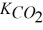Try out our new practice tests completely free!# Quiz 6: SolutionsThe label on a jar of jam says it contains 13 g of sucrose per tablespoon (15 mL).What is the molarity of sucrose in the jam? [Molar mass: sucrose,342.3 g/mol]
(Multiple Choice)An unopened soda bottle at 25°C contains CO2 gas at 6.2 atm.Calculate the equilibrium concentration of CO2 in the unopened soda bottle.[= 3.1 × 10-2 mol/(L•atm)]
(Multiple Choice)What is the boiling point of a 1.5 m solution of NaCl? [kb = 0.52°C/m]
(Multiple Choice)Cardiac arrest victims are sometimes treated by injection of a calcium chloride solution directly into the heart muscle.If 2.0 mL of a 5.0% (m/V)CaCl2 solution is administered to a patient,what mass of CaCl2 was provided?
(Multiple Choice)A solution in equilibrium with undissolved solute is said to be unsaturated.
(True False)A chemist needs 0.725 moles of acetic acid for a particular reaction.What volume of a 1.50 M acetic acid solution is needed to provide this amount?
(Multiple Choice)If a solvent is colorless,all of its true solutions will also be colorless.
(True False)Which statement is FALSE?
(Multiple Choice)The solubility of KBr at 20°C is 60 g/100 g H2O.Increasing the temperature of the solution will likely increase the solubility of KBr.
(True False)Which statement concerning solutions is FALSE?
(Multiple Choice)Which statement concerning solution concentration is TRUE?
(Multiple Choice)What is the molarity of a solution prepared by diluting 10.0 mL of 18.0 M HCl with enough water to make 500.0 mL of solution?
(Multiple Choice)The solid material that separates from solution when its solubility is exceeded is called a precipitate.
(True False)What is the molarity of a solution that contains 4.50 mol of NaF dissolved in 750.0 mL of solution?
(Multiple Choice)An unknown amount of water is added to 75 mL of a 3.5 M sodium chloride solution.What can be said for certain about the concentration of the solution that results?
(Multiple Choice)In normal room lighting,the eye cannot distinguish a true solution from a colloidal one.
(True False)One liter of alcohol combined with one liter of water does NOT produce two liters of solution.
(True False)An alloy,such as brass,is an example of a solution in the solid state.
(True False)Which statement concerning solutions is FALSE?
(Multiple Choice)A patient requires 100.0 g of glucose in the next 12 hours.What volume of a 5.0% (m/V)glucose solution should be administered?
(Multiple Choice)## Filters

• Essay(0)
• Multiple Choice(0)